# Polyhedral Compounds

Steven Dutch, Professor Emeritus, Natural and Applied Sciences, University of Wisconsin - Green Bay

## Uniform Polyhedra and Their Duals

Each of the Platonic and Kepler-Poinsot polyhedra can be combined with its dual. There are five possibilities:

• Tetrahedron with itself
• Cube and Octahedron
• Icosahedron and Dodecahedron
• Great Dodecahedron and Small Stellated Dodecahedron
• Great Icosahedron and Great Stellated Dodecahedron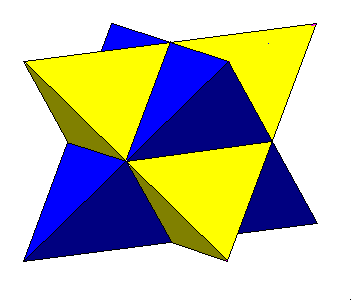Stella Octangula The tetrahedron is its own dual. Two interpentrating tetrahedra make up the stella octangula, discovered by Kepler. Its vertices are the vertices ofd a cube, its edges are the face diagonals of a cube, and the solid common to both tetrahedra is an octahedron. This is also one of the regular compounds discussed below.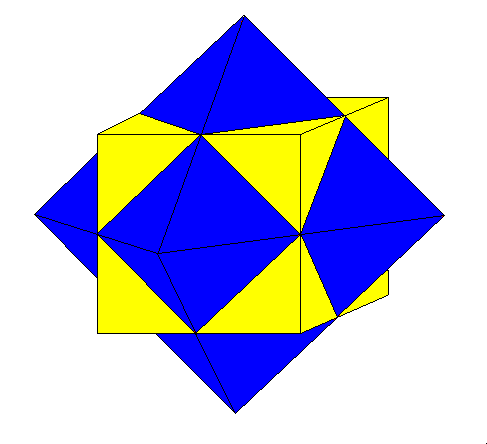Cube and Octahedron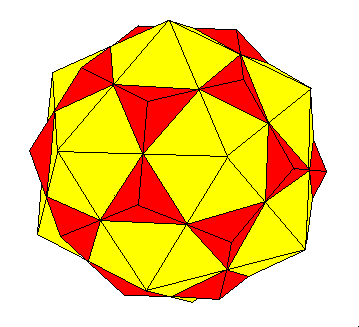Icosahedron and DodecahedronGreat Dodecahedron and Great Stellated Dodecahedron

## Regular Compounds

In addition to combining polyhedra and their duals, there are several regular compounds of polyhedra that result in solids with greater symmetry than the component polyhedra. These are all based on the fact that some Platonic solids can be inscribed in others. There are five possibilities of which one has already been presented:

• Tetrahedra in a cube (Stella Octangula, above)
• Cubes in a dodecahedron
• Octahedra around an icosahedron
• Five tetrahedra in a dodecahedron
• Ten Tetrahedra in a dodecahedron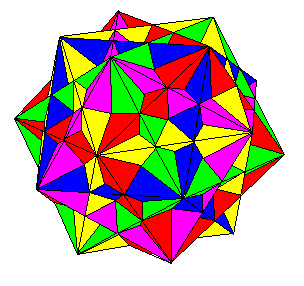Five Cubes in a Dodecahedron A dodecahedron contains the vertices of a cube. There are five different ways to inscribe a cube in a dodecahedron; each diagonal of a pentagon is one possible cube edge. Superposition of all five cubes results in a solid with icosahedral symmetry.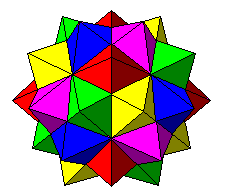Five Octahedra Around an Icosahedron The icosahedron and octahedron are duals of the dodecahedron and cube. This compound is the dual of the five-cube compound. An icosahedron contains the faces of an octahedron; there are five different ways to inscribe an icosahedron in an octahedron. Superposition of all five octahedra results in a solid with icosahedral symmetry. Since this shape results from extending the faces of an icosahedron, it is one of the stellations of the icosahedron.Five Tetrahedra in a Dodecahedron A tetrahedron can be inscribed within a cube, or obtained by extending alternate faces of an octahedron. Thus, either of the two compounds above can derive this compound of five tetrahedra. This shape lacks mirror planes and thus comes in either left- or right-handed form. Since this shape can result from extending the faces of the octahedra above, it therefore also results from extending the faces of an icosahedron and is thus one of the stellations of the icosahedron.

## Other Scaled Compounds of Uniform polyhedra

There are many other interesting and attractive compounds of polyhedra. In some cases the relative sizes of the intersecting polyhedra are fixed by the intersections of vertices and edges. I refer to these as "scaled". Of course, compounds of identical polyhedra are scaled. In other cases the relative sizes are not fixed. I refer to those as "nonscaled"Crossed Dodecahedra (Iron Cross) A cube can be inscribed in a dodecahedron but a dodecahedron does not have cubic symmetry. We can inscribe a cube in two dodecahedra, rotate one of them 90 degrees, and superimpose them to get a compound with full cubic symmetry. Another way to describe this shape is to say that we rotate one of the two dodecahedra 90 degrees around one of its two-fold symmetry axes. You cannot have repeating plane patterns with five-fold symmetry, so crystals can never be true pentagonal dodecahedra. However, the mineral pyrite often crystallizes as slightly distorted dodecahedra, and sometimes two crystals interpenetrate to make the shape shown here. A crystal of pyrite with this form is sometimes called an "iron cross".Crossed Icosahedra We can also combine two icosahedra by rotating one 90 degrees around one of its two-fold symmetry axes and superimposing them. Eight pairs of faces are coplanar and result in skewed six-pointed stars.Three Cubes Three cubes are rotated 45 degrees around their four-fold symmetry axes, then superimposed.Four Cubes - 1 This compound rotates each of four cubes around one of its diagonal three-fold symmetry axes. The compound has full cubic symmetry but the four-fold symmetry axes do not line up with those of the component cubes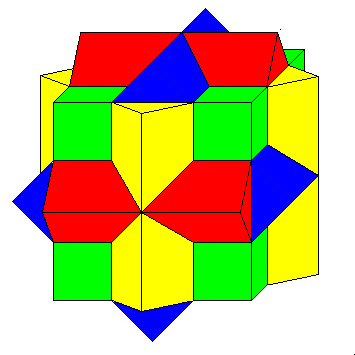Four Cubes - 2 Here three cubes are rotated around their four-fold axes, and a fourth non-rotated cube added. The faces are 8/2 polygons, usually considered degenerate polygons because their edges do not form a continuous set. 8/2 star polygons are popular decorative motifs in the Islamic world.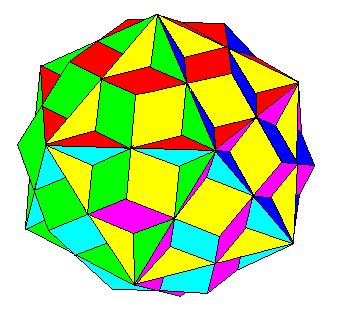Great Dodecahedron and Dodecahedron A compound of the great dodecahedron and a regular dodecahedron.Octahedron and Dodecahedron - 1 The cube inscribed in a dodecahedron cannot be seen from outside except for its edges, which are coplanar with the dodecahedron faces. Its dual octahedron, however, protrudes through the dodecahedron.Octahedron and Dodecahedron - 2 A slightly larger octahedron just touches the opposite vertices of the dodecahedron, so that the edges of the octahedron are completely visible.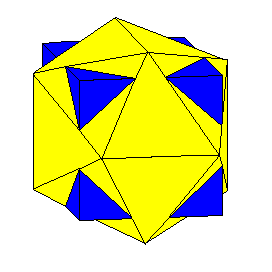Icosahedron and Cube An icosahedron encloses an octahedron. A cube oriented with the same symmetry as the octahedron can interpenetrate the icosahedron.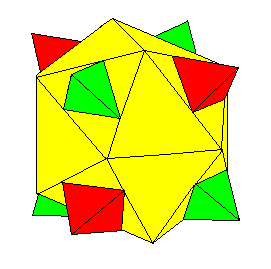Icosahedron and two tetrahedraIcosahedron and single tetrahedron

## Nonscaled Compounds of Uniform polyhedra

These compunds mostly show how polyhedra are related; the relative sizes of the interpenetrating polyhedra can vary within limits without affecting the symmetry of the compound.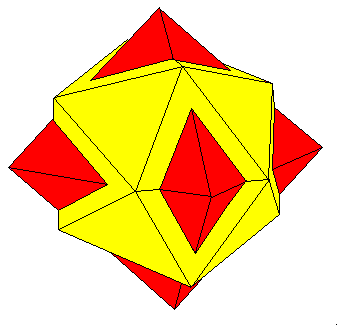Octahedron and Icosahedron This compound illustrates how an octahedron is related to an icosahedron.

Created 5 December 1997, Last Update 7 June 1999